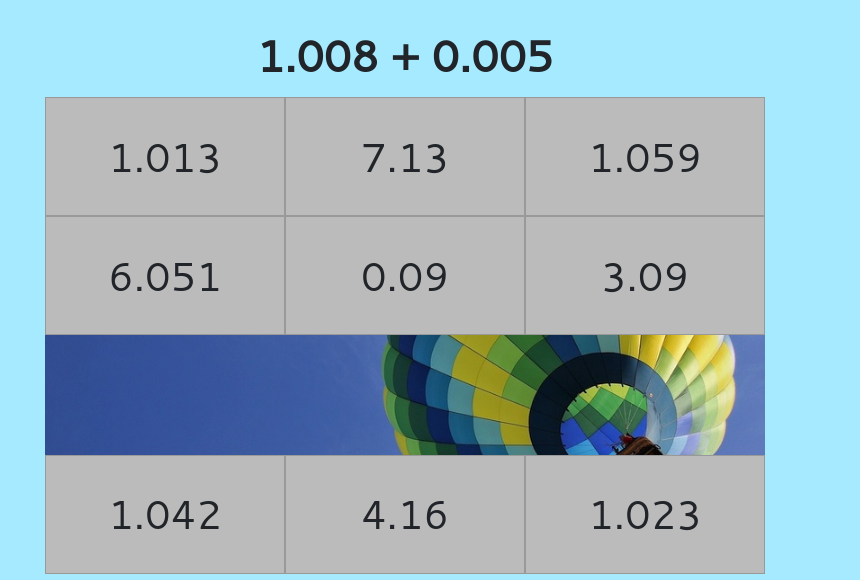MM Practice
×
Multiplication
Division
Place Value
Fractions & Decimals
Measurement
Statistics & Probability
Pre-Algebra
Money
Kindergarten
Geometry

# Decimal Addition & Subtraction Hidden Picture Game - online practice (grades 4-7)Online practice for 4th-7th grade

Solve decimal addition and subtraction problems while uncovering a hidden picture in this fun game!

The problems encourage the use of mental math and can be solved in one's mind using strategies based on place value. For example, you might see 0.006 + 0.7 or 1.2 − 0.05.

This decimal game is most useful for students in grades 4-7.

Screenshot:"Correct" sound credit: http://www.orangefreesounds.com